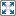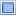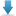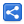# Capturing vs Non-Capturing Lambdas

[Last Updated: Feb 10, 2016]

A lambda expression is said to be capturing if it either access instance variables of it's enclosing class or local variables (final or effectively final) from it's enclosing scope.

A non-capturing lambda doesn't use any variables from outside.

Non-capturing lambdas generally are considered more efficient than the capturing ones as they are evaluated only once.

Let's do some quick tests on different scenarios and see which one performs better.

#### Lambda capturing local final variables:

```     final BigDecimal bd = new BigDecimal(1);
Function<BigDecimal, BigDecimal> func = (a) -> bd.multiply(a);

for (int j = 0; j < 999999999; j++) {
func.apply(new BigDecimal(j));
}```

Average time taken: 1725 ms

#### Lambda capturing local effectively final variables:

```      BigDecimal bd = new BigDecimal(1);
Function<BigDecimal, BigDecimal> func = (a) -> bd.multiply(a);

for (int j = 0; j < 999999999; j++) {
func.apply(new BigDecimal(j));
}```

Average time taken: 1688 ms

#### Lambda instance variable capturing

```public class LambdaInstanceCapturing implements Runnable {

private BigDecimal bd = new BigDecimal(1);

@Override
public void run() {
Function<BigDecimal, BigDecimal> func = (a) -> bd.multiply(a);

for (int j = 0; j < 999999999; j++) {
func.apply(new BigDecimal(j));
}
}
}```

Average time taken: 1750 ms

#### Non-capturing Lambda

In this example we are just multiplying the passed BigDecimal variable with constant `BigDecimal.TEN`. It's still not accessing anything from outside as static final constants are inlined by the compiler .

```   Function<BigDecimal, BigDecimal> func = (a) -> a.multiply(BigDecimal.TEN);

for (int j = 0; j < 999999999; j++) {
func.apply(new BigDecimal(j));
}```

Average time taken: 1651 ms

#### Conclusion:

Non-capturing Lambda is performing little better than others. But I won't suggest to make decisions based on that as things are changing with each minor release of Java 8. It's generally good to know all aspects of lambdas though.

#### Example Project

Dependencies and Technologies Used:

• JDK 1.8
• Maven 3.0.4

 Java Capturing Lambda TestSelect AllDownload• capturing-lambda-tests
• src
• main
• java
• com
• logicbig
• example
• MainBenchmarkTest.java

The class MainBenchmarkTest runs each test 5 times and output the average.

Output:

```Lambda local final capturing  time: 1725
Lambda local effectively final capturing  time: 1688
Lambda instance capturing  time: 1750
Lambda non capturing  time: 1651
```

The Java version I used

```c:\>java -version
java version "1.8.0_65"
Java(TM) SE Runtime Environment (build 1.8.0_65-b17)
Java HotSpot(TM) 64-Bit Server VM (build 25.65-b01, mixed mode)
```
System info:
```C:\>systeminfo
...
OS Name:                   Microsoft Windows 8.1
OS Version:                6.3.9600 N/A Build 9600
OS Configuration:          Standalone Workstation
OS Build Type:             Multiprocessor Free
.....
System Type:               x64-based PC
Processor(s):              1 Processor(s) Installed.
: Intel64 Family 6 Model 71 Stepping 1 GenuineIntel ~2701 Mhz
....
Total Physical Memory:     16,299 MB
Available Physical Memory: 8,893 MB
Virtual Memory: Max Size:  18,752 MB
Virtual Memory: Available: 9,204 MB
Virtual Memory: In Use:    9,548 MB
```

 Share